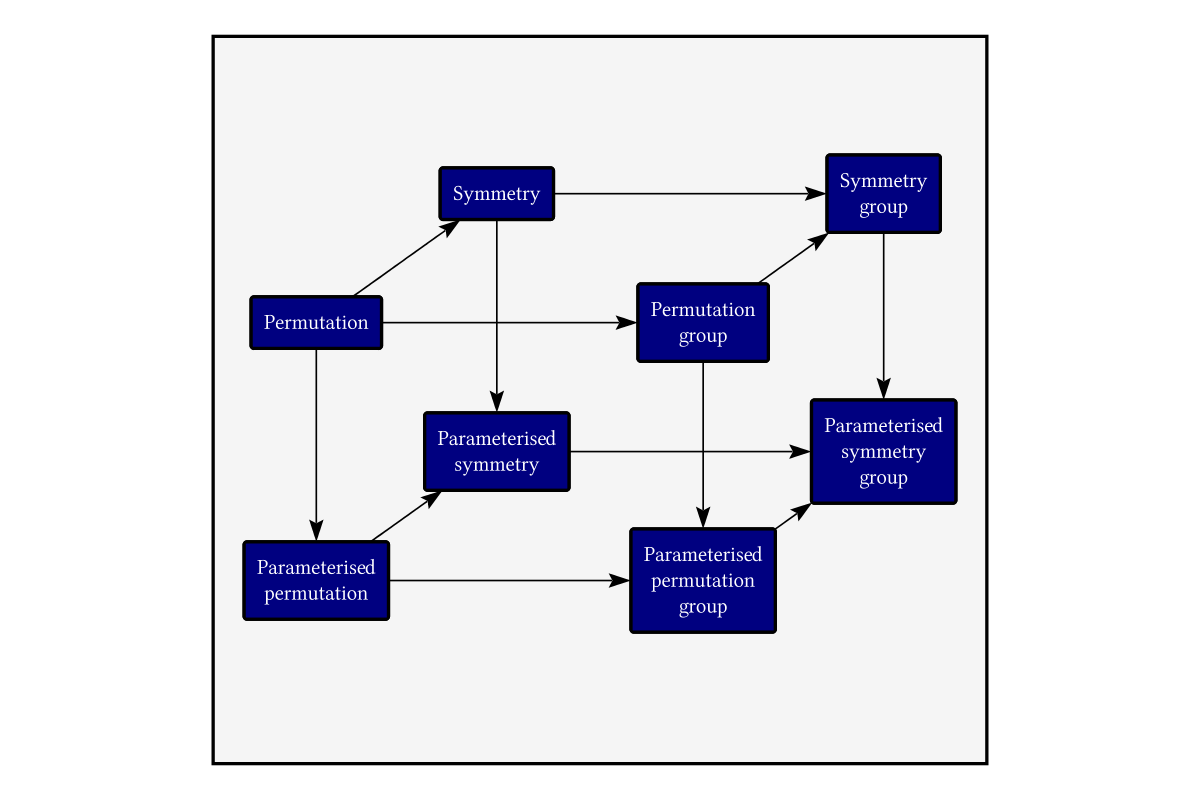Basic diagram with text, boxes and arrows.Author: Chris Mears
> import Diagrams.Backend.SVG.CmdLine
> import Data.List.Split
> import Data.Maybe
> import Diagrams.BoundingBox
> import Diagrams.Prelude
> import Graphics.SVGFonts

The diagram is the boxes (the “cube”) and the lines between the boxes.

> example = let c = sCube
>           in pad 1.1 . centerXY $c <> drawLines c <> square 30 > # fc whitesmoke > # scaleY 0.94 > # translateX 11 > # translateY (-3) A “box” is a diagram (the “innards”) surrounded by a rounded rectangle. First the innards are padded by a fixed amount, then we compute its height and width – that’s the size of the surrounding rectangle. > box innards padding = > let padded = strutY padding > === > (strutX padding ||| centerXY innards ||| strutX padding) > === > strutY padding > height = diameter (r2 (0,1)) padded > width = diameter (r2 (1,0)) padded > in centerXY innards <> roundedRect width height 0.1 > > textOpts n = TextOpts lin2 INSIDE_H KERN False 1 n A single string of text. > text' :: String -> Double -> Diagram B > text' s n = textSVG_ (textOpts n) s # fc white # lw none Several lines of text stacked vertically. > centredText ls n = vcat' (with & catMethod .~ Distrib & sep .~ n) > (map (\l -> centerX (text' l n)) ls) > centredText' s = centredText (splitOn "\n" s) Diagram-specific parameters, including the positioning vectors. > padAmount = 0.5 > > down = r2 (0, -10) > > upright = r2 (7, 5) > > right = r2 (15, 0) A box with some interior text and a name. > mybox s n = (box (centredText' s 1) padAmount) # named n The cube is just several boxes superimposed, positioned by adding together some positioning vectors. > sCube :: Diagram B > sCube = fc navy$ mconcat
>   [ mybox "Permutation" "perm"
>   , mybox "Permutation\ngroup" "permgroup"                     # translate right
>   , mybox "Symmetry" "sym"                                     # translate upright
>   , mybox "Parameterised\npermutation" "paramperm"             # translate down
>   , mybox "Parameterised\npermutation\ngroup" "parampermgroup" # translate (right ^+^ down)
>   , mybox "Parameterised\nsymmetry" "paramsym"                 # translate (down ^+^ upright)
>   , mybox "Symmetry\ngroup" "symgroup"                         # translate (upright ^+^ right)
>   , mybox "Parameterised\nsymmetry\ngroup" "paramsymgroup"     # translate (down ^+^ right ^+^ upright)
>                ]

For each pair (a,b) of names, draw an arrow from diagram “a” to diagram “b”.

> drawLines :: Diagram B -> Diagram B
> drawLines cube = foldr (.) id (map (uncurry
>                        (connectOutside' (with
>                        & shaftStyle %~ lw thin))) pairs) cube
>   where pairs = [ ("perm","permgroup")
>                 , ("perm","sym")
>                 , ("perm","paramperm")
>                 , ("paramperm","paramsym")
>                 , ("sym","symgroup")
>                 , ("paramsym","paramsymgroup")
>                 , ("permgroup","symgroup")
>                 , ("paramperm","parampermgroup")
>                 , ("symgroup","paramsymgroup")
>                 , ("sym","paramsym")
>                 , ("permgroup","parampermgroup")
>                 , ("parampermgroup","paramsymgroup")
>                 ]
> main = mainWith (example :: Diagram B)